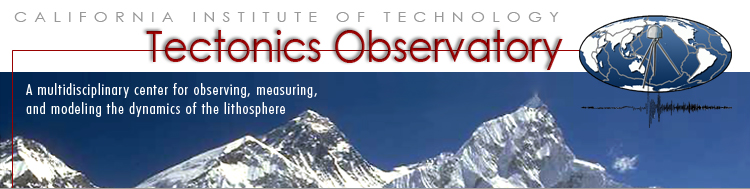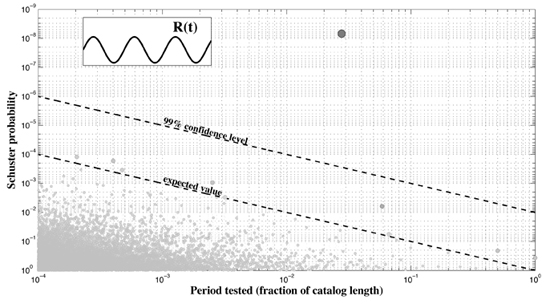Schuster Spectrum - searching for periodicities in the timing of earthquakes

 This software can be used to search for periodicities in the timing of earthquakes. The software takes a catalog of earthquake events (such as the timings of earthquakes in Nepal), as well an upper and lower bound on the period, and from these computes and plots a Schuster spectrum. First the program "Schuster_spectrum.m" computes the subset of periods to be considered (these lie between the two bounding periods). Then, for each of these periods, the program "Schuster_test_log.m" performs a Schuster test on the catalog, i.e., it looks at how much the timing of events in the catalog correlates with each periodicity, and computes the probability of observing such a level of correlation by chance (called the Schuster p-value). The lower the Schuster p-value, the more likely the catalog exhibits that periodicity. The program then plots the spectrum of Schuster p-values, and as well as the significant detection levels. Download software: Schuster_spectrum.m Schuster_Test_Log.mSchuster spectrum for a synthetic 1000-event earthquake catalog generated by using a harmonically varying seismicity rate (inset shows schematic of rate). The time unit for the period tested is the total duration of the catalog. The period-dependent "expected value" dashed line represents the minimum p-value expected for a catalog that has no periodicity. p-values that reach the 99% confidence level identify periodicities; in this case there is one such period.